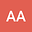•The goal of this paper is to investigate the geometry of the warping function on a $n$-dimensional compact Legenderian warped product submanifold $M^n$ of Sasakian space form with free boundary. We establish sharp estimates to the squared norm of the second fundamental form and the Laplacian of the warping function. Besides, we provide some triviality results for $M^n$ by using the Ricci curvature along the gradient of the warping function. Taking the clue from the Bochner formula and second-order ordinary differential equation, we find the characterization for the base of $M^n$ via the first non-zero eigenvalue of the warping function and proved that it is isometric to Euclidean space $\mathbb{R}^p$ or Euclidean sphere $\mathbb{S}^p$ under some extrinsic conditions.